# Nodal Analysis problem with voltage sources

## Homework Statement

I have a circuit and I need to find the Nodal Equations that would mathematically describe the circuit, then proceed to finding the voltages at each node using Cramers rule and the currents at nodes 2 and 4. I dont think I would have much of a problem utilising Cramers rule. The problem I have right now is deriving the Nodal Equations.
However I would love to have the rest of my methods validated since I am simply unsure if I am on the right track or not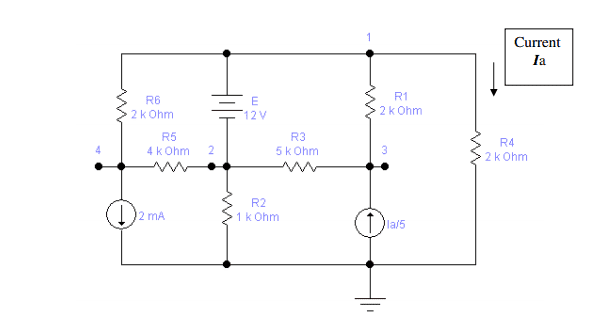## The Attempt at a Solution

I am quite sure I have many errors in my attempted solution at deriving equations to describe the circuit. I am unsure what to do with the voltages sources.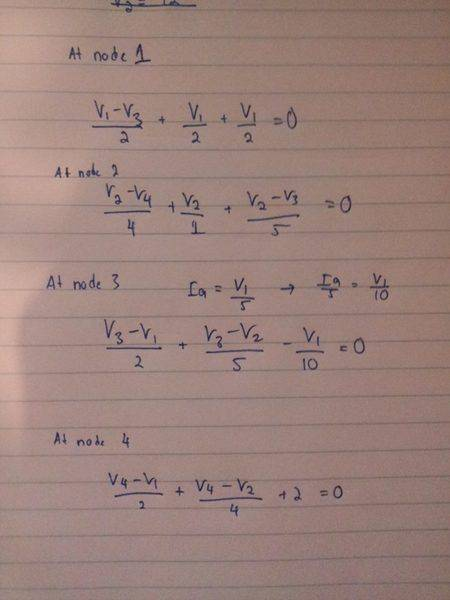Please find the diagram of the circuit and my attempt at solving the problem in the attached files.

Thank you very much in advance!

gneill
Mentor
The 12V battery fixes the potential between your nodes 1 and 2, so they don't constitute independent essential nodes. In fact, the two taken together comprise a "supernode".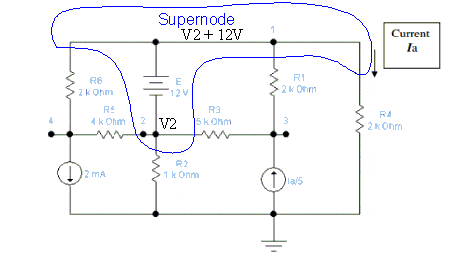Keep only one variable to describe the potential for the supernode (V1 or V2) and write a single node equation. Suppose you keep V2 as the variable for the supernode, then the potential at the former node 1 connections would be "V2+12".

#### Attachments

•1 person
So I take the super node and consider it as a combined node consisting of node 1 and node 2?

Here is my new system of equations and the voltages I got from each node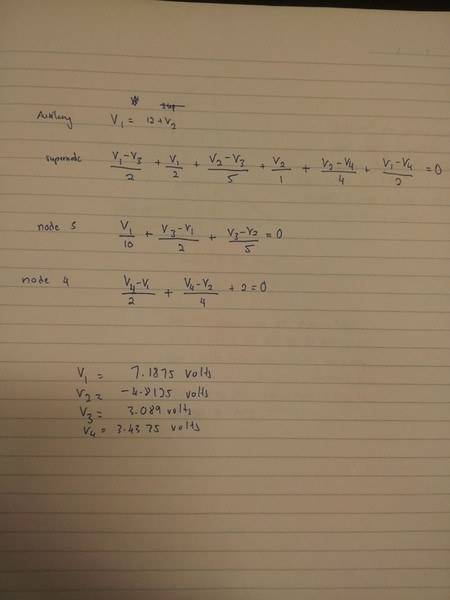I am unsure if it correct or not as the values I calculated for V3 do not seem to be very attractive.

I realised I made a mistake at node 3, It should be -V1/10.

Anyways here are the new voltages I came up with

V1 = 8.214
V2 =-3.7857
V3 = 6.57
V4 = 4.4

I am completely unsure on how I can find the current at node 2 and 4 and show KCL holds for each though...

Do I just find the nodal equation for node 2 and node 4 and find the current using the voltages that I have already derived earlier to find the total current in each node?

gneill
Mentor
I realised I made a mistake at node 3, It should be -V1/10.
Yup, I spotted that and was going to mention it when I thought I'd check this later postYour equations, with that update, now look perfect.
Anyways here are the new voltages I came up with

V1 = 8.214
V2 =-3.7857
V3 = 6.57
V4 = 4.4
Strangely enough, your previous set of numbers look closer to what I'm getting... Must be some slips creeping into the mechanics of solving the equations. Are you using Cramer's Rule or just substitutions?

•1 person
Yup, I spotted that and was going to mention it when I thought I'd check this later postYour equations, with that update, now look perfect.

Strangely enough, your previous set of numbers look closer to what I'm getting... Must be some slips creeping into the mechanics of solving the equations. Are you using Cramer's Rule or just substitutions?

I was using cramers rule. I did spot another error that I made. I forgot to multiply the 2 by 4 when trying to eliminate the denominators to form the matrice.

So anyways here are my new set of solutions which I believe look much better

V1= 7.14 or 50/7
V2= -4.857 or -34/7
V3= 4.734 or 232/49
V4= 0.476 or 10/21

gneill
Mentor
I was using cramers rule. I did spot another error that I made. I forgot to multiply the 2 by 4 when trying to eliminate the denominators to form the matrice.

So anyways here are my new set of solutions which I believe look much better

V1= 7.14 or 50/7
V2= -4.857 or -34/7
V3= 4.734 or 232/49
V4= 0.476 or 10/21

Much better indeed!Thank you very much for your time and help!

Unfortunately I still have another question
"Using the voltages at each node calculate the current for nodes 2 & 4 and show Kirchhoff’s Current Law (KCL) holds for each of these nodes."

I think I am mostly having trouble interpreting this question but if I am not mistaken I am just subbing the values V1-V4 obtained back into the equations found at the nodes? If this is the case I am unsure how I can attempt to solve for Node 2.

Thanks again!

gneill
Mentor
Thank you very much for your time and help!
I'm happy to help!
Unfortunately I still have another question
"Using the voltages at each node calculate the current for nodes 2 & 4 and show Kirchhoff’s Current Law (KCL) holds for each of these nodes."

I think I am mostly having trouble interpreting this question but if I am not mistaken I am just subbing the values V1-V4 obtained back into the equations found at the nodes? If this is the case I am unsure how I can attempt to solve for Node 2.
Yes, you'll use the voltage values that you obtained for the nodes and plug them into the appropriate terms of the node equations to find the individual current values.

I agree that node 2 is a bit trickier since there's no explicit term in your equations corresponding to the current through the battery. However, if you consider the supernode as a whole, the current through its "battery leg" can be found using KCL. Does that make sense?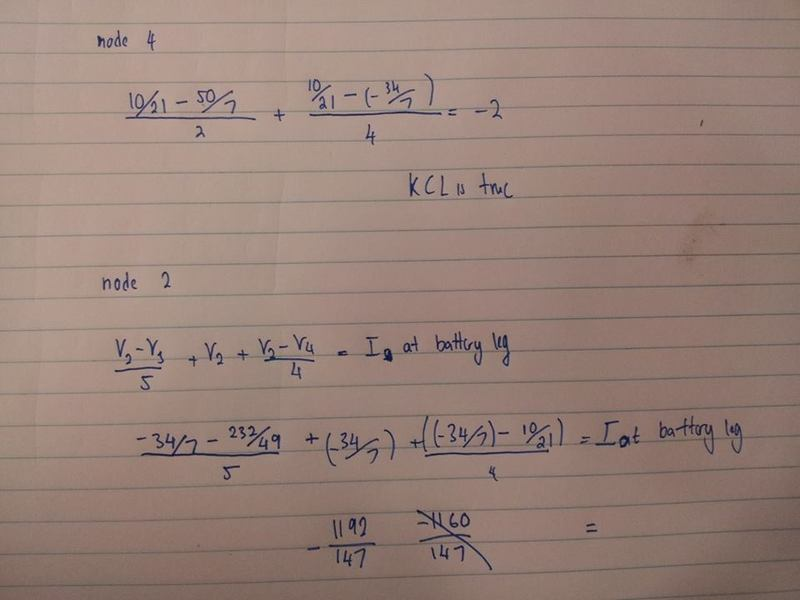So for Node 4 I am just essentially showing that the currents through the resistors entering the node is equal to the current found at the current source.

As for node 2, I am showing that the current at the battery leg connected to node 2 is equal to the currents through the resistors connected to node 2?

gneill
Mentor
View attachment 62094

So for Node 4 I am just essentially showing that the currents through the resistors entering the node is equal to the current found at the current source.
Sure. The idea being to show that the sum of all currents entering and leaving a given node is zero.
As for node 2, I am showing that the current at the battery leg connected to node 2 is equal to the currents through the resistors connected to node 2?

Take a step back from node 2 to begin with and find the current in the battery leg first. To do that, consider the supernode as a whole. It has a total of four connections to the rest of the network, one of which is that battery leg. KCL to find the battery current.

Then use that battery current to show that it satisfies the KCL requirement for your node 2 equation.

Really, this part of the problem is a bit sneaky since while it seems at first glance to be a small task involving four currents, it actually forces you to calculate a lot more currents than just those at node 2!

yeah......ok you lost me there :S.

I cant figure out which is the four connections?

gneill
Mentor
yeah......ok you lost me there :S.

I cant figure out which is the four connections?

Oops. My bad. I'd forgotten that node 2 is actually part of the supernode!Consider instead node 1 alone. It has the four connections you need to consider.

The Electrician
Gold Member
Another way to deal with voltages sources without considering "supernodes", is to use Modified Nodal Analysis:

http://www.swarthmore.edu/NatSci/ech.../mna/MNA2.html [Broken]

MNA will give the current through the voltage source directly. For your problem, the MNA formulation in matrix form would be, with the source current denoted IE: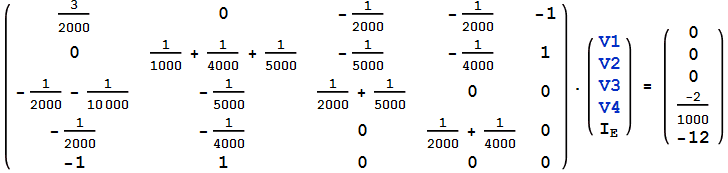Solving the system, we get: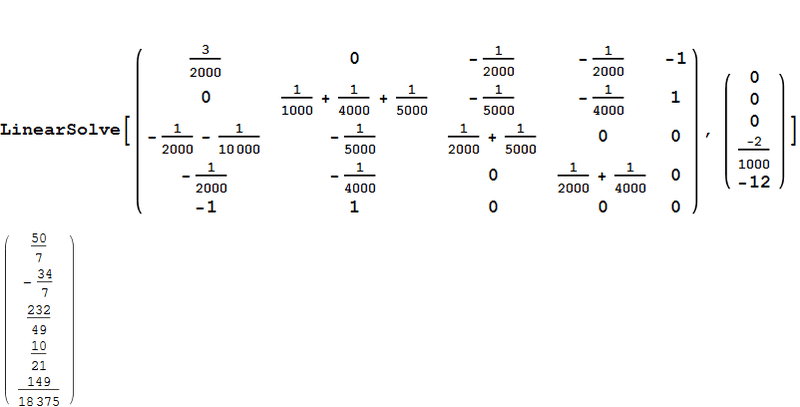The current in the source is 149/18375 amps.

#### Attachments

Last edited by a moderator:
Another way to deal with voltages sources without considering "supernodes", is to use Modified Nodal Analysis:

http://www.swarthmore.edu/NatSci/ech.../mna/MNA2.html [Broken]

MNA will give the current through the voltage source directly. For your problem, the MNA formulation in matrix form would be, with the source current denoted IE: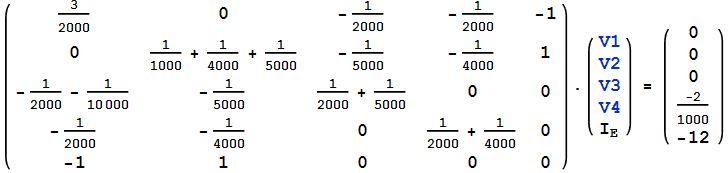Solving the system, we get: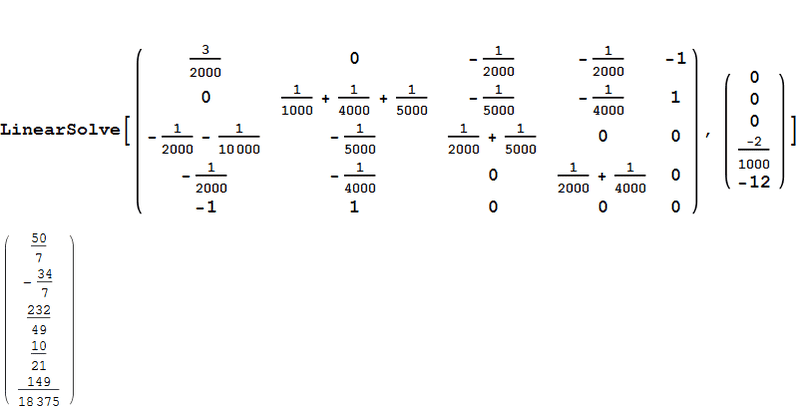The current in the source is 149/18375 amps.

I would use MNA but a 5x5 matrice is not very attractive...

Last edited by a moderator:
Oops. My bad. I'd forgotten that node 2 is actually part of the supernode!Consider instead node 1 alone. It has the four connections you need to consider.

If I were to just consider those 4 connections, wouldn't that mean the current would just be the minus of what I obtained in my solution? or should the values be the one posted using the MNA method?

The Electrician
Gold Member
Without using MNA, you could get the current in the source by knowing the current in R1, R4 and R6. Then you will have all the currents at node 2, which you can show all sum to zero.

You already got all the node voltages in post #7; I only showed the MNA solution as an example of an alternate method to get the current in the source directly.